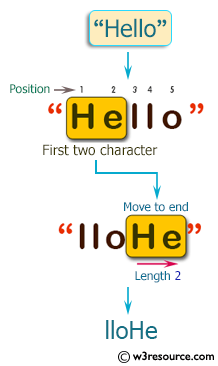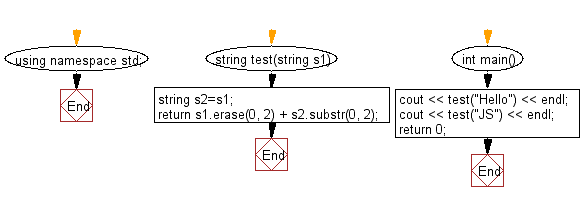﻿ C++ : Add the first two characters to the end of a string# C++ Exercises: Move the first two characters to the end of a given string of length at least two

## C++ Basic Algorithm: Exercise-65 with Solution

Write a C++ program to move the first two characters to the end of a given string of length at least two.

Sample Solution:

C++ Code :

``````#include <iostream>
using namespace std;
string test(string s1)
{
string s2=s1;
return s1.erase(0, 2) + s2.substr(0, 2);
}
int main()
{
cout << test("Hello") << endl;
cout << test("JS") << endl;
return 0;
}
``````

Sample Output:

```lloHe
JS
```

Pictorial Presentation:Flowchart:C++ Code Editor: